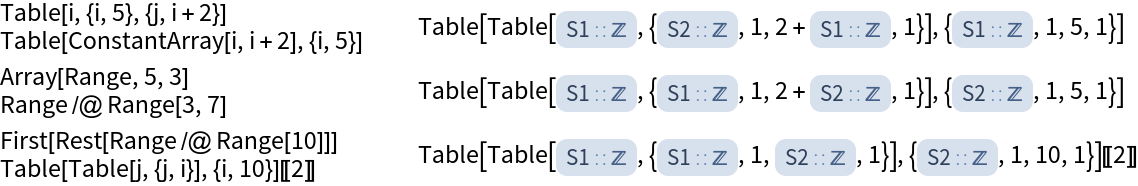# Wolfram/CodeEquivalenceUtilities

Utilities for testing code equivalence

Contributed By: Richard Hennigan

CodeEquivalenceUtilities is a collection of Wolfram Language functions that can be used to test if different pieces of code are equivalent without the need for evaluation.

This allows comparison of unevaluated expressions that may have non-deterministic outputs (e.g. random values, dates, etc).

This Paclet represents the underlying technology that powers several automated code grading systems, such as the online exercises for EIWL and Wolfram Challenges.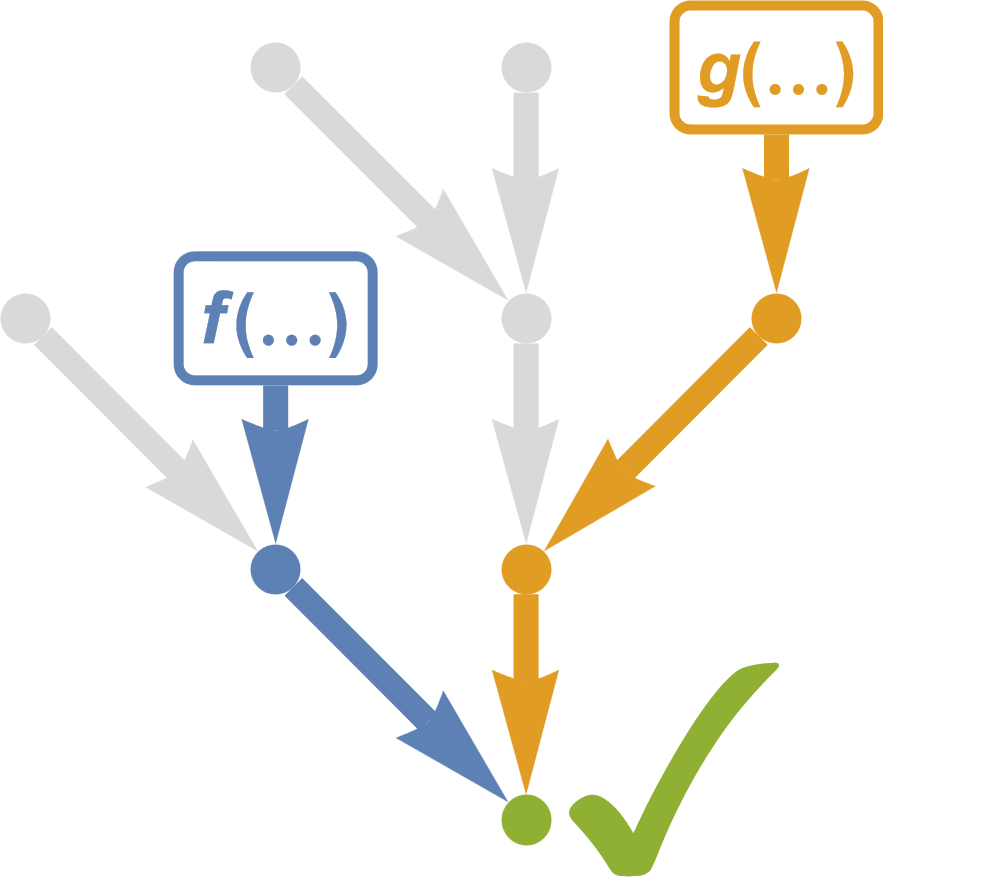## Installation Instructions

To install this paclet in your Wolfram Language environment, evaluate this code:
PacletInstall[ResourceObject["Wolfram/CodeEquivalenceUtilities"]]

## Examples

### Basic Examples (2)

Check if two expressions are equivalent:

 In:=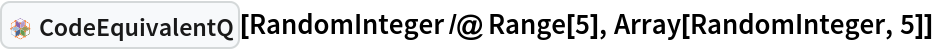Out=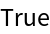View the canonical representations of expressions:

 In:=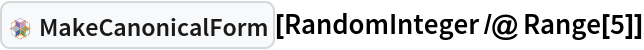Out=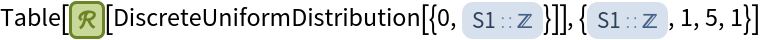In:=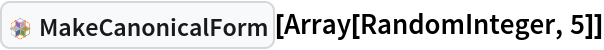Out=These are directly comparable:

 In:=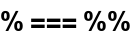Out=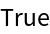### Scope (3)

 In:=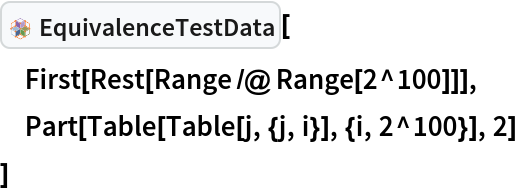Out=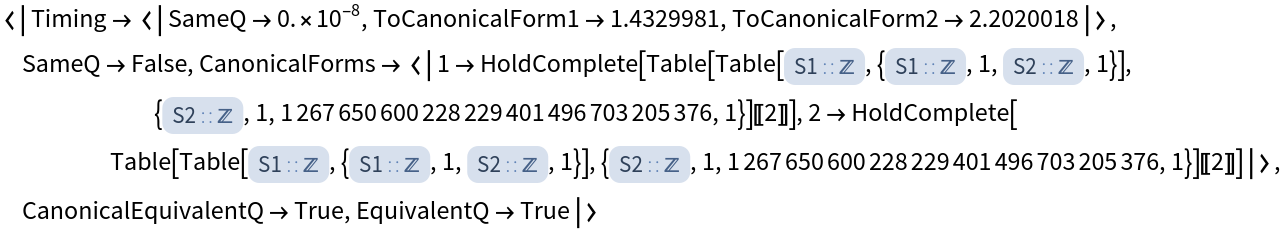View the sequence of transformations used to convert an expression to its canonical form:

 In:=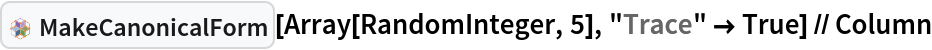Out=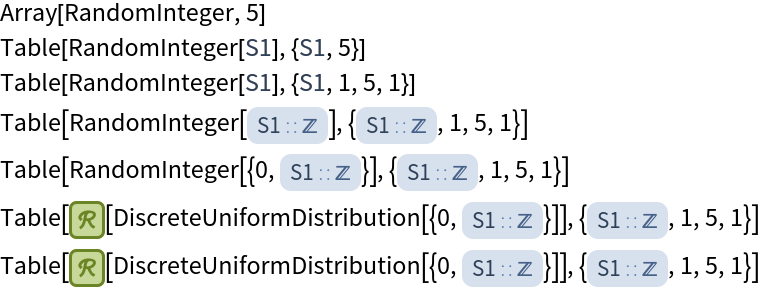Convert a canonical representation to a normal expression:

 In:=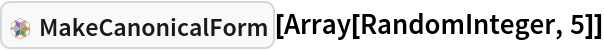Out=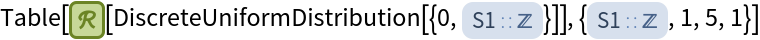In:=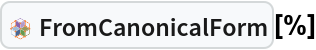Out=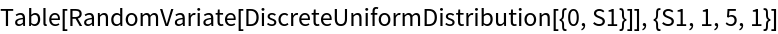Evaluate:

 In:=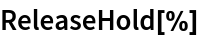Out=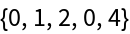### Neat Examples (6)

Here is a list of expressions, some of which are equivalent to others:

 In:=Find the sequence of transformations for each expression:

 In:=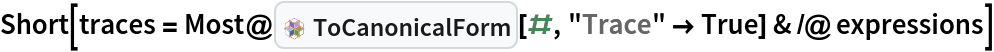Out=Generate a graph for each sequence:

 In:=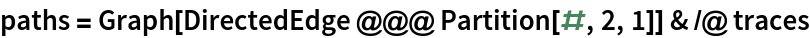Out=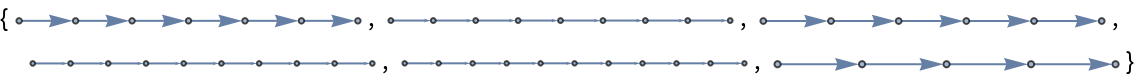Combine the graphs:

 In:=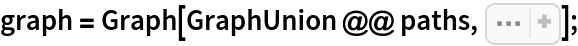Equivalent expressions converge to the same connected component:

 In:=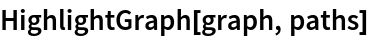Out=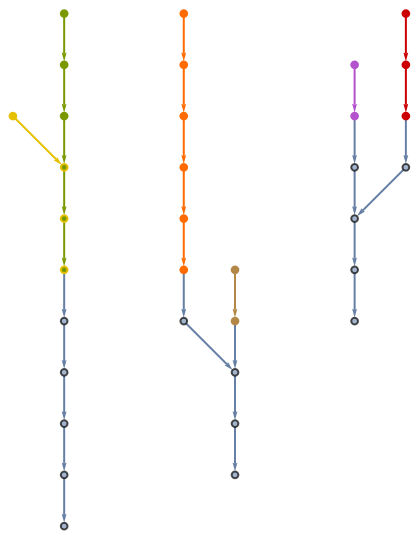Group the expressions into their corresponding equivalence class:

 In:=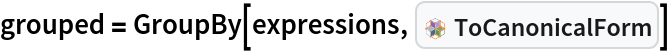Out=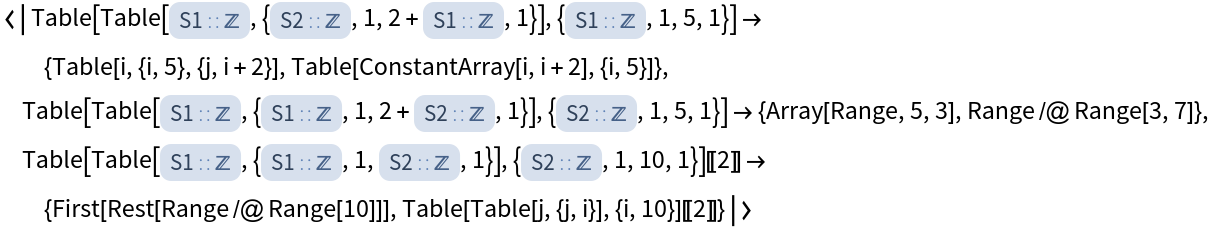In:=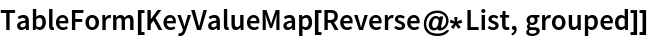Out=